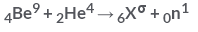Courses

# Test: Nuclear Energy

## 10 Questions MCQ Test Physics Class 12 | Test: Nuclear Energy

Description
This mock test of Test: Nuclear Energy for JEE helps you for every JEE entrance exam. This contains 10 Multiple Choice Questions for JEE Test: Nuclear Energy (mcq) to study with solutions a complete question bank. The solved questions answers in this Test: Nuclear Energy quiz give you a good mix of easy questions and tough questions. JEE students definitely take this Test: Nuclear Energy exercise for a better result in the exam. You can find other Test: Nuclear Energy extra questions, long questions & short questions for JEE on EduRev as well by searching above.
QUESTION: 1

### Artificial disintegration with alpha particles of which of the following led to the discovery of neutron?

Solution:

James Chadwick set up an experiment to test his hypothesis. Chadwick put a piece of beryllium in a vacuum chamber with some polonium. The polonium emitted alpha rays, which struck the beryllium. When struck, the beryllium emitted the mysterious neutral rays.

QUESTION: 2

### In a nuclear reactor:

Solution:

A moderator is used in nuclear reactors in order to slow down the speed of neutrons.
In nuclear engineering, a neutron moderator is a medium that reduces the speed of fast neutrons, thereby turning them into thermal neutrons capable of sustaining a nuclear chain reaction involving uranium-235 or a similar fissile nuclide.
Clearly, an obvious way to make a reactor work, and to make use of this characteristic of the 235U(n,f) cross-section, is to slow down the fast, fission neutrons. This can be accomplished, for example, when the neutrons collide with nuclei and scatter in some substance (a moderator).

QUESTION: 3

### What is the binding energy of the hydrogen nucleus?​

Solution:

Nucleus of hydrogen has only one proton. Hence its binding energy will be zero. The nuclear binding energy is the energy it takes to separate all the nucleons in a nucleus from each other. Since there is only the one nucleon, it's already separated from any other nucleons.

QUESTION: 4

The fusion of hydrogen into helium is more likely to take place:

Solution:

The fusion of hydrogen into helium is more likely to take place at high temperature and high pressure, like the sun.

QUESTION: 5

Given M = mass of the nucleus, A = atomic mass. What is packing fraction?

Solution:

Packing fraction: - It tells about the stability of a nucleus.
Packing fraction=isotonic mass=molecular mass (atomic mass)/atomic mass
p.f.=M-A/A

QUESTION: 6

Why does the fusion occur at high temperature?

Solution:

Fusion reactions takes place at temperature about 107K. It requires this high temperature so that nucleus are moving at very high speed, so that they have high kinetic energy and can overcome the repulsion between nuclei and come together.

QUESTION: 7

What amount of energy is released in the fission of 95U235 ?​

Solution:

The fission process represented by the equation, 92​U235+0​n156​Ba144+36​Kr89+30​n1
Masses of reactants =234.39+1.01=235.4amu
Masses of products =143.28+88.89+3(1.01) =235.2amu
Energy released = mass difference =235.4−235.2=0.2amu=0.2×931∼200MeV

QUESTION: 8

In the following reactionWhat is following value of a?​

Solution:

The sum of the atomic no. and atomic mass no. on the reactant and product should be equal .
therefore 9+4 = a+1
a = 12

QUESTION: 9

Which of the following statements is true for nuclear forces?

Solution:

Nuclear forces are short range forces. This is the only correct answer. Others are wrong. They are the strongest forces in nature and do not obey inverse square law.

QUESTION: 10

A fusion bomb involves:​

Solution:

Nuclear fusion is the process by which two or more atomic nuclei join together, or “fuse,” to form a single heavier nucleus. During this process, matter is not conserved because some of the mass of the fusing nuclei is converted to energy, which is released.